Join the forum, it's quick and easy

Would you like to react to this message? Create an account in a few clicks or log in to continue.

# 〔問題〕交通標線

3 posters##〔問題〕交通標線Lasoireearx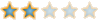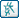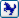##先看看大概是不是這樣畫

Option Explicit

Public tm As AcadModelSpace ' 設 tm 簡化名稱圖紙空間
Public pi As Double

Public Sub markline_drawing()

On Error Resume Next

ThisDrawing.SendCommand "ucs" & vbCr & "w" & vbCr
ThisDrawing.SendCommand "undo" & vbCr & "be" & vbCr
pi = 3.141592 / 180

Set tm = ThisDrawing.ModelSpace: Set tu = ThisDrawing.Utility

Dim origin_p(0 To 2) As Double
Dim p1, p2, p3, p4 As Variant
Dim start_p As Variant
Dim points(0 To 9) As Double
Dim line_p1 As Variant
Dim line_p2 As Variant
Dim markline_length As Double
Dim i_count As Integer
Dim markline_number As Integer
Dim dis As Integer
Dim markline_angle As Single

line_p1 = tu.GetPoint(, "請點選標線起點, ESC 結束 !")

If Err Then Exit Sub

line_p2 = tu.GetPoint(line_p1, "請點選標線終點, ESC 結束 !")

If Err Then Exit Sub

dis = 400 + 600

markline_length = Int(line_obj.Length)
markline_number = Int(markline_length / dis)
markline_angle = line_obj.Angle

'MsgBox markline_length & Space(3) & markline_number

For i_count = 1 To markline_number

start_p = tu.PolarPoint(line_p1, markline_angle, (i_count - 1) * dis)

p1 = tu.PolarPoint(start_p, markline_angle + 90 * pi, 10)
p2 = tu.PolarPoint(p1, markline_angle + 0, 400)
p3 = tu.PolarPoint(p2, markline_angle + 270 * pi, 20)
p4 = tu.PolarPoint(p3, markline_angle + 180 * pi, 400)

' Define the 2D polyline points
points(0) = start_p(0): points(1) = start_p(1)
points(2) = p1(0): points(3) = p1(1)
points(4) = p2(0): points(5) = p2(1)
points(6) = p3(0): points(7) = p3(1)
points(8) = p4(0): points(9) = p4(1)

Set lw_pline = tm.AddLightWeightPolyline(points): lw_pline.Closed = True: lw_pline.Color = 2: lw_pline.Update

Next i_count

ThisDrawing.SendCommand "undo" & vbCr & "e" & vbCr

End Subshackle_2005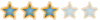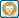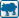##回復： 〔問題〕交通標線Lasoireearx##回復： 〔問題〕交通標線naruto018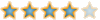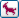HEMOS likes this post

##回復： 〔問題〕交通標線Lasoireearx##回復： 〔問題〕交通標線shackle_2005